# English and math

1/5 of a class study English, and 3/5 study mathematics. If the class consist of 80 students, how many students study English and mathematics in the class?

E =  16
M =  48

### Step-by-step explanation:Did you find an error or inaccuracy? Feel free to write us. Thank you!Tips to related online calculators
Need help to calculate sum, simplify or multiply fractions? Try our fraction calculator.

## Related math problems and questions:

• ShirtsIn a classroom, 1/6 of the students are wearing blue shirts and 2/3 are wearing white shirts. There are 36 students in the class. How many students are wearing a shirt other than blue or white?
• Pupils 7There are 40 pupils in a certain class. 3/5 of the class are boys. How many are girls?
• There 11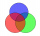There are 50 pupil in the class. Out of of this number,1/10 speak French only and 4/5 of the 10 remainder speak both French and English . If the rest speak English only find the number of students who speak only English?
• Math classificationIn 3A class are 27 students. One-third got a B in math, and the rest got an A. How many students received a B in math?
• 5 2/55 2/5 hours a week  mathematics,  3 3/4 hours a week   Natural sciences, 4 3/8 hours a week  Technology . how many hours does  he spend on social sciences if he spend 17 1/2 hours a week for the four subject?
• Shirts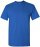In a classroom,1/6 of the students are wearing blue shirts, and 2/3 are wearing white shirts. There are 18 students in the classroom. How many students are wearing shirts other than blue shirts or white shirts?
• Evaluate expressionEvaluate expression using BODMAS rule: 1 1/4+1 1/5÷3/5-5/8
• Students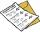The class has 22 students. 2 of which has assesement of 2. Count of assesement of 1 is three times more than count of students with assesement of 3. How many students has assesement of 1 and how many students has assesement of 1.
• Venn diagram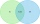University students chose a foreign language for the 1st year. Of the 120 enrolled students, 75 chose English, 65 German, and 40 both English and German. Using the Venn diagram, determine: - how many of the enrolled students chose English only - how many
• Class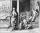When Pythagoras asked how many students attend the school, he said: "Half of the students studying mathematics, 1/4 music, seventh silent, and there are three girls at school." How many students had Pythagoras at school?
• Lunch timeIn a cafeteria, 3/10 of the students are eating salads, and 3/5 are eating sandwiches. There are 30 students in the cafeteria. How many students are eating lunches other than salads or sandwiches?
• Math testIn mathematics, there were 25 problems of three kinds: light 2 points, medium 3 points, heavy 5 points, the best score is 84 points. How many points did Jane have when she solved all the easy examples, half medium and one-third difficult?
• Fractions and mixed numerals(a) Convert the following mixed numbers to improper fractions. i. 3 5/8 ii. 7 7/6 (b) Convert the following improper fraction to mixed number. i. 13/4 ii. 78/5 (c) Simplify these fractions to their lowest terms. i. 36/42 ii. 27/45 2. evaluate following ex
• The studyJames was preparing for a quiz. He studied 5/21 hours on Saturday and 2/7 hours on Sunday. How many hours did he study over the weekend?
• Fraction of a NumberIf 1/2 of 1/3 of 1/4 of 1/5 of a number is 2.5. what is the number?
• Diamonds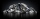If simva had 8 diamond and cuts them each into 1/3 pieces, then how many pieces does she have?
• The ratioThe ratio of girls to boys in Mrs. White's class is 3:2.  If there are 12 boys, how many girls are in the class room?